DOC HOME SITE MAP MAN PAGES GNU INFO SEARCH PRINT BOOK
Floating point operations

# Denormalized numbers

A number is denormalized if the exponent field contains all 0's and the fraction field does not contain all 0's.

Thus denormalized single-precision numbers can be in the range (plus or minus)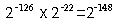to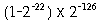inclusive.

Denormalized double-precision numbers can be in the range (plus or minus)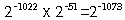to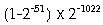inclusive.

Denormalized extended-precision numbers do not have a 1 bit in position 63. Therefore, it stores numbers in the range (plus or minus)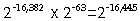to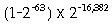inclusive.

Both positive and negative zero values exist, but they are treated the same during floating point calculations.

Next topic: Maximum and minimum representable floating point values
Previous topic: Normalized numbers